##### Trigonometry Workbook For DummiesSometimes, when you are subtracting large numbers, the top digit in a column is smaller than the bottom digit in that column. In that case, you need to borrow from the next column to the left. Borrowing is a two-step process:
1. Subtract 1 from the top number in the column directly to the left.

Cross out the number you’re borrowing from, subtract 1, and write the answer above the number you crossed out.

2. Add 10 to the top number in the column you were working in.

For example, suppose you want to subtract 386 – 94. The first step is to subtract 4 from 6 in the ones column, which gives you 2: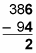When you move to the tens column, however, you find that you need to subtract 8 – 9. Because 8 is smaller than 9, you need to borrow from the hundreds column. First, cross out the 3 and replace it with a 2, because 3 – 1 = 2: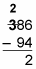Next, place a 1 in front of the 8, changing it to an 18, because 8 + 10 = 18: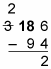Now you can subtract in the tens column: 18 – 9 = 9: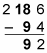The final step is simple: 2 – 0 = 2: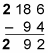Therefore, 386 – 94 = 292.

In some cases, the column directly to the left may not have anything to lend. Suppose, for instance, you want to subtract 1,002 – 398. Beginning in the ones column, you find that you need to subtract 2 – 8. Because 2 is smaller than 8, you need to borrow from the next column to the left. But the digit in the tens column is a 0, so you can’t borrow from there because the cupboard is bare, so to speak: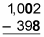When borrowing from the next column isn’t an option, you need to borrow from the nearest non-zero column to the left.

In this example, the column you need to borrow from is the thousands column. First, cross out the 1 and replace it with a 0. Then place a 1 in front of the 0 in the hundreds column: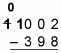Now, cross out the 10 and replace it with a 9. Place a 1 in front of the 0 in the tens column: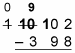Finally, cross out the 10 in the tens column and replace it with a 9. Then place a 1 in front of the 2: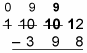At last, you can begin subtracting in the ones column: 12 – 8 = 4: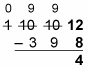Then subtract in the tens column: 9 – 9 = 0: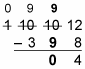Then subtract in the hundreds column: 9 – 3 = 6: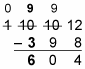Because nothing is left in the thousands column, you don’t need to subtract anything else. Therefore, 1,002 – 398 = 604.